# ACS Sustainable Chem. Eng. | 利用新型牛磺酸基咪唑离子液体处理木质纤维素生物质直接生产乙酰丙酸乙酯

2022
12/14
15:57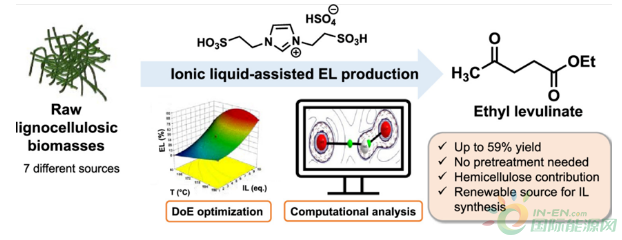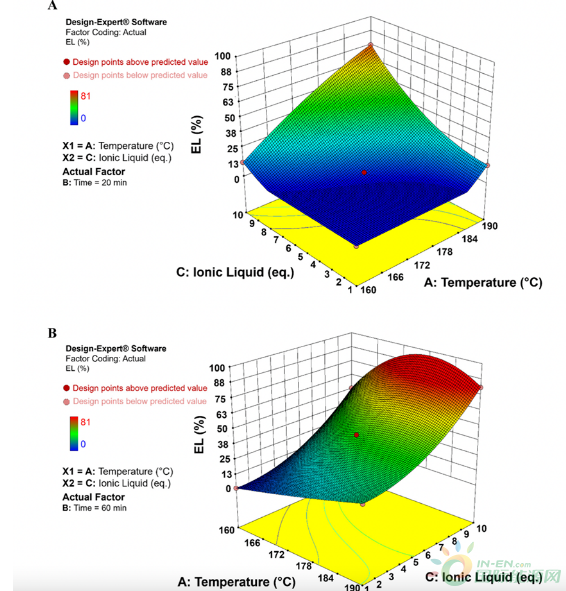Fig. 1. Response surfaces for EL production from microcrystalline cellulose as a function of temperature and IL (equiv) when time is fixed at (A) 20 min and (B) 60 min.

Understanding the Molecular Interactions by Computational Studies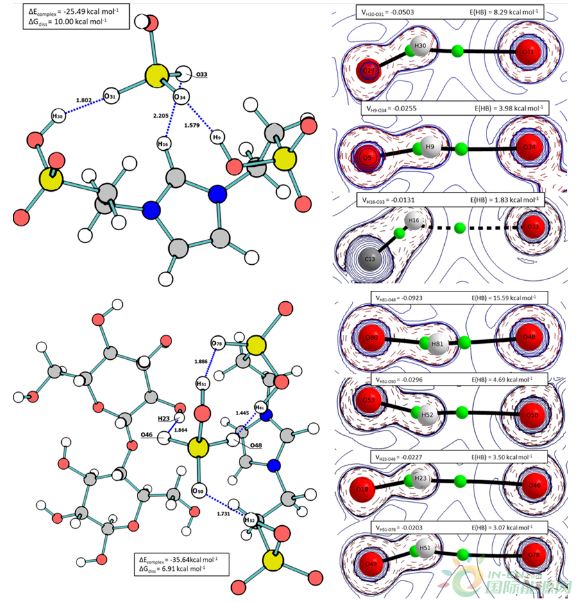Fig. 2.  (a) Intermolecular complex between taurine-derived imidazolium cation and hydrogen sulfate anion ([TauIm][HSO4]) observed at 190°C. Interaction energies were calculated using QTAIM calculations by applying the equation EHB (V) = 0.277|V|?0.45. Thermodynamic properties were obtained by DFT calculations (M06-2X/6-31++(2d,p)). Dashed lines represent the observed hydrogen bond interactions (hydrogen bond distance values given in angstroms).  (b) Intermolecular complex between [TauIm][HSO4] and cellobiose observed at 190°C. Interaction energies were calculated using QTAIM calculations by applying the equation EHB (V) = 0.277|V| – 0.45. Thermodynamic properties were obtained by DFT calculations (M06-2X/6-31++(2d,p)). Dashed lines represent the observed hydrogen bond interactions (hydrogen bond distance values given in angstroms).

EL Production from Lignocellulosic Biomasses Mediated by [TauIm][HSO4]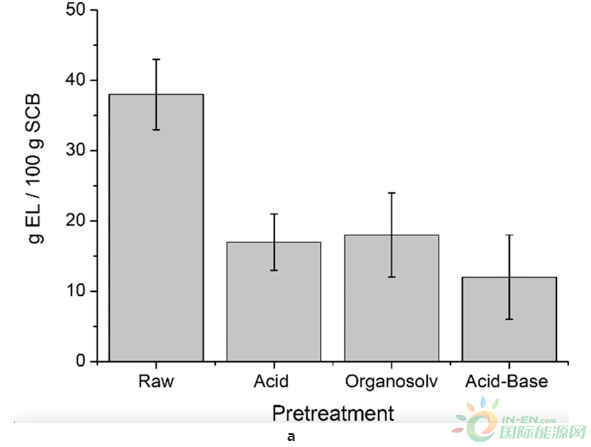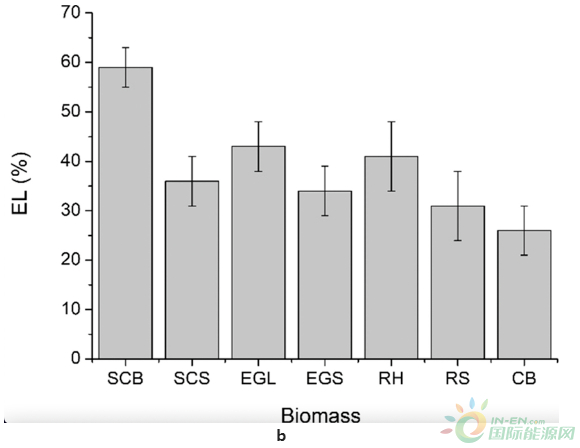Fig. 3. （a) Mass EL yields (g EL/ 100 g SCB) from raw and pretreated SCBs. (b) EL production from different lignocellulosic materials.